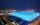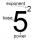Volume and area

What is the volume of a cube which has area of 361 cm2?

Result

V =  466.696 cm3

Solution:Leave us a comment of example and its solution (i.e. if it is still somewhat unclear...):Be the first to comment!To solve this example are needed these knowledge from mathematics:

Tip: Our volume units converter will help you with converion of volume units.

Next similar examples:

1. Cube volumeThe cube has a surface of 384 cm2. Calculate its volume.
2. Cube 6Surface area of one wall cube is 1600 cm square. How many liters of water can fit into the cube?
3. Area to volumeIf the surface area of a cube is 486, find its volume.
4. Calculate 3Calculate the cube volume whose edge is 3x-1,3x-1,3x-1
5. Cube 1-2-3Calculate the volume and surface area of the cube ABCDEFGH if: a) /AB/ = 4 cm b) perimeter of wall ABCD is 22 cm c) the sum of the lengths of all edges of the cube is 30 cm.
6. Cube wallsFind the volume and the surface area of the cube if the area of one of its walls is 40 cm2.
7. Sphere VSFind the surface and volume of a sphere that has a radius of 2 dm.
8. Cube 7Calculate the volume of a cube, whose sum of the lengths of all edges is 276 cm.
9. Edges or sidesCalculate the cube volume, if the sum of the lengths of all sides is 276 cm.
10. InscribedCube is inscribed in the cube. Determine its volume if the edge of the cube is 10 cm long.
11. Volume of cubeSolve the volume of a cube with width 26cm .
12. Cube 8The surface of the cube is 0.54 m2. Calculate the length of the cube edge.
13. Cube wallSurface of first cube wall his 225 mm2. Second cube area is 60% of the surface of the first cube. Determine the length of the edge of the second cube (x).
14. Cube edgeDetermine the edges of the cube when the surface is equal to 37.5 cm square.
15. CubeThe sum of all cube edges is 30cm. Find the surface area of the cube.
16. Unknown number 17Milada said, I am thinking of a number such that I evaluate expression x1/3, the value of the expression would be 5. Which number Milada thinking?
17. Simplify 2Simplify expression: 5ab-7+3ba-9